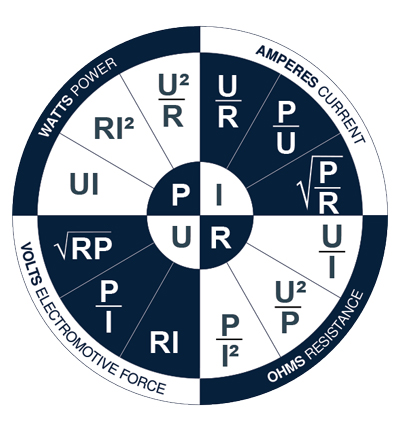# FormulasOhm’s Law: Valid only for DC circuits

U = R x I
P = U x I

3 Phased AC power for electrical motor

P = U x I x √3 x cos Ф

U: Voltage (V), R: Resistance (Ohm), I: Current (A), P: Power (W)